# 5. Suppose Y represents a single observation from the probability density function given by: Soyo-1, 0,...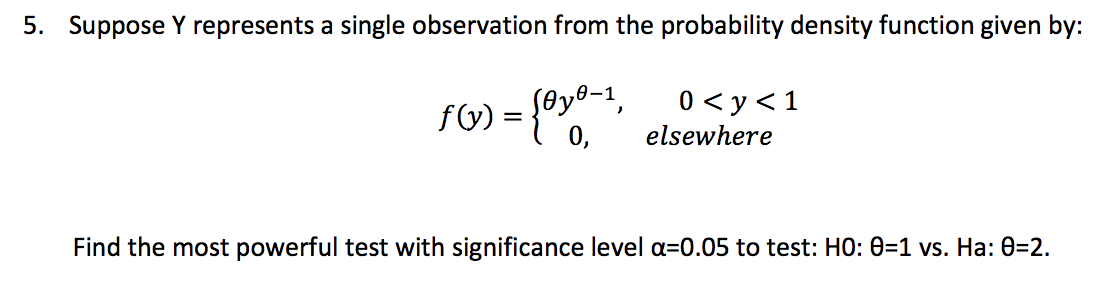5. Suppose Y represents a single observation from the probability density function given by: Soyo-1, 0, 0<y<1 elsewhere Find the most powerful test with significance level a=0.05 to test: HO: 0=1 vs. Ha: 0=2.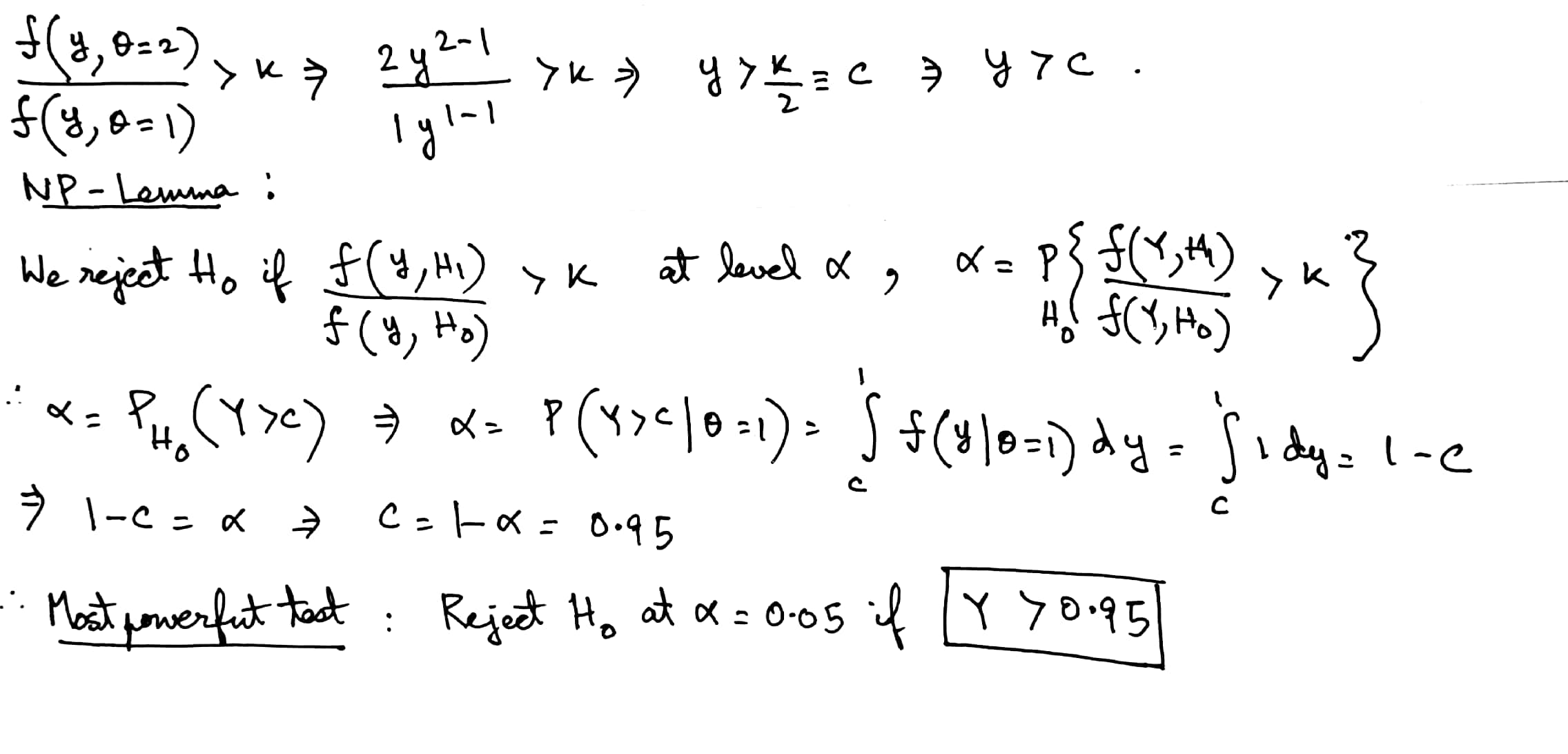##### Add Answer to: 5. Suppose Y represents a single observation from the probability density function given by: Soyo-1, 0,...
Similar Homework Help Questions
• ### If x is a single observation taken from population has probability density function fx(x,0)-28x +...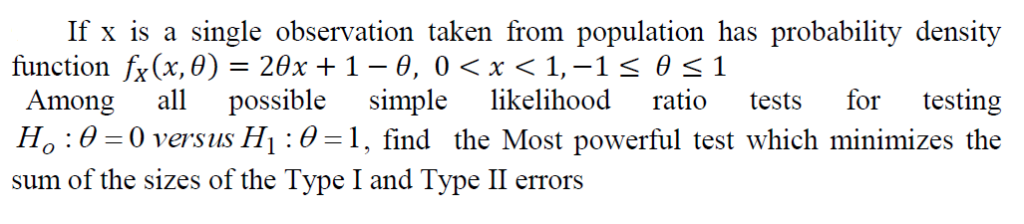i need the solution with steps If x is a single observation taken from population has probability density function fx(x,0)-28x + 1-0, 0 < x < 1,-1 θ 1 Among all possible simple likelihood ratio tests for testing s the Ho:0 0 versus H:0-1, find the Most powerful test which sum of the sizes of the Type I and Type II errors If x is a single observation taken from population has probability density function fx(x,0)-28x + 1-0, 0

• ### 1. Consider the joint probability density function 0<x<y, 0<y<1, fx.x(x, y) = 0, otherwise. (a) Find...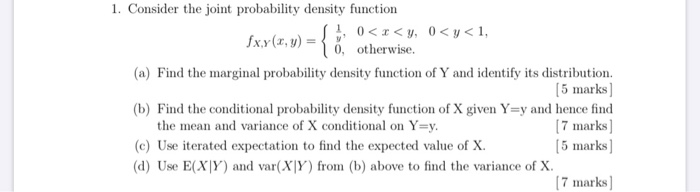1. Consider the joint probability density function 0<x<y, 0<y<1, fx.x(x, y) = 0, otherwise. (a) Find the marginal probability density function of Y and identify its distribution. (5 marks (b) Find the conditional probability density function of X given Y=y and hence find the mean and variance of X conditional on Y=y. [7 marks] (c) Use iterated expectation to find the expected value of X [5 marks (d) Use E(XY) and var(XY) from (b) above to find the variance of...

• ### You have observed one observation X from a distribution with probability density function fx (x) ...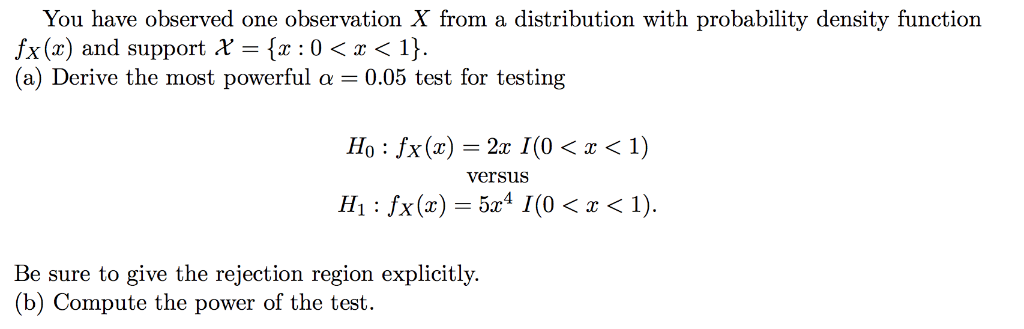You have observed one observation X from a distribution with probability density function fx (x) and support X = {x : 0 〈 x 〈 1} (a) Derive the most powerful α 0.05 test for testing Ho : fx(x) = 2x 1 (0 < x < 1) versus H1 : fx (x) = 5c4 1 (0 〈 x 〈 1). Be sure to give the rejection region explicitly. (b) Compute the power of the test You have observed one observation...

• ### Find the probability that Y is greater than 3. Let Y have the probability density function...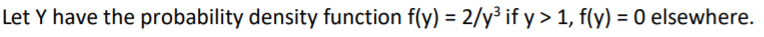Find the probability that Y is greater than 3. Let Y have the probability density function f(y) = 2/y3 if y> 1, f(y) = 0 elsewhere.

• ### Q. Suppose the joint probability density function of X and Y is (a) Show that the...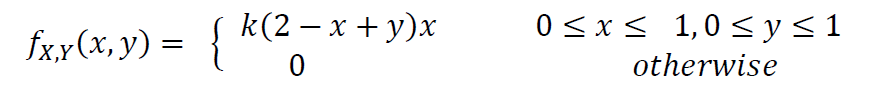Q. Suppose the joint probability density function of X and Y is (a) Show that the value of constant ?=12/11 (b) Find the marginal density function of X, i.e., fX(x). (c) Find the conditional probability density of X given Y = y, i.e., fX|Y(x|y). fxy(x, y) = s k(2 - x + y)x 1 0 0 < x < 1,0 = y = 1 otherwise

• ### 1 1 Let X be a single observation from a population with density function 0-e- for x = 0, 1, 2, ,...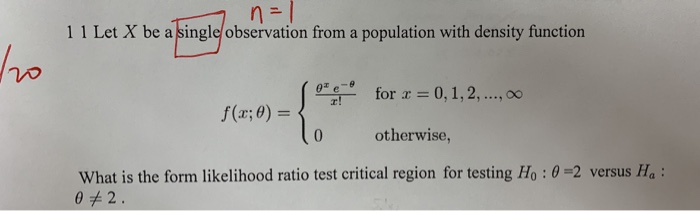1 1 Let X be a single observation from a population with density function 0-e- for x = 0, 1, 2, ,00 0 otherwise, What is the form likelihood ratio test critical region for testing Ho : θ-2 versus Ha : 1 1 Let X be a single observation from a population with density function 0-e- for x = 0, 1, 2, ,00 0 otherwise, What is the form likelihood ratio test critical region for testing Ho : θ-2 versus...

• ### 7. Show that if the joint probability density function of X and Y is if 0...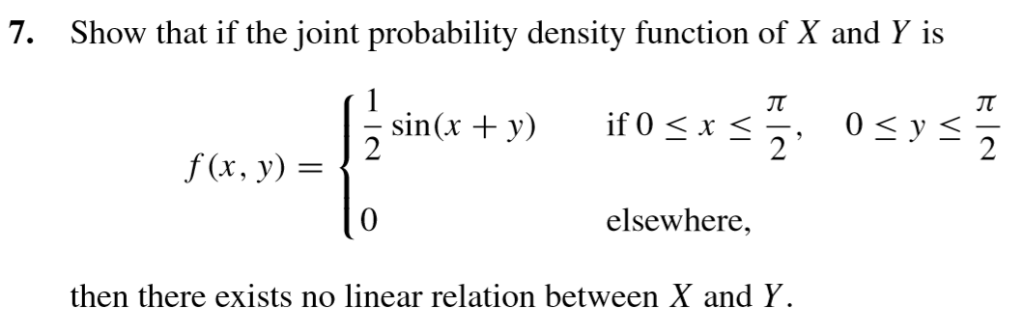7. Show that if the joint probability density function of X and Y is if 0 < x <.. =sin(x + y) f(x, y) = { VI fres 9 Line + »» Hosszž, osys elsewhere, then there exists no linear relation between X and Y.

• ### If x is a single observation taken from population has probability density Among possible simple ...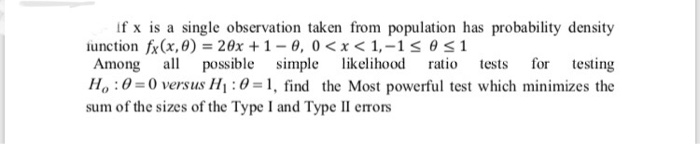i need the solution with steps if x is a single observation taken from population has probability density Among possible simple likelihood ratio tests for testing Ho : θ 0 versus HI :6-1, find the Most powerful test which minimizes the sum of the sizes of the Type I and Type II erors if x is a single observation taken from population has probability density Among possible simple likelihood ratio tests for testing Ho : θ 0 versus HI :6-1,...

• ### Suppose a joint probability density function for two variables X and Y is given as follows:...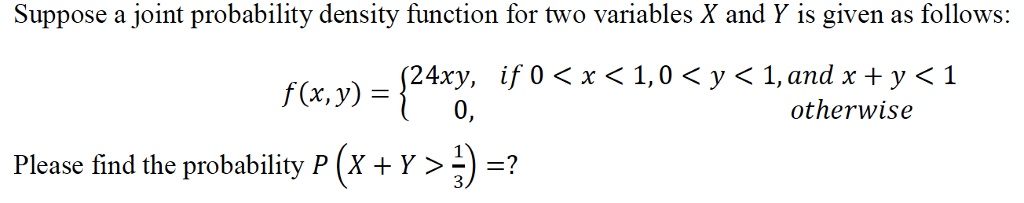Suppose a joint probability density function for two variables X and Y is given as follows: {24x0, if 0 < x < 1,0 < y < 1 f(x, y) = otherwise Please find the probability p (w > 1) =? 3

• ### 4. Let X and Y have joint density function le-x 0 < y < x <...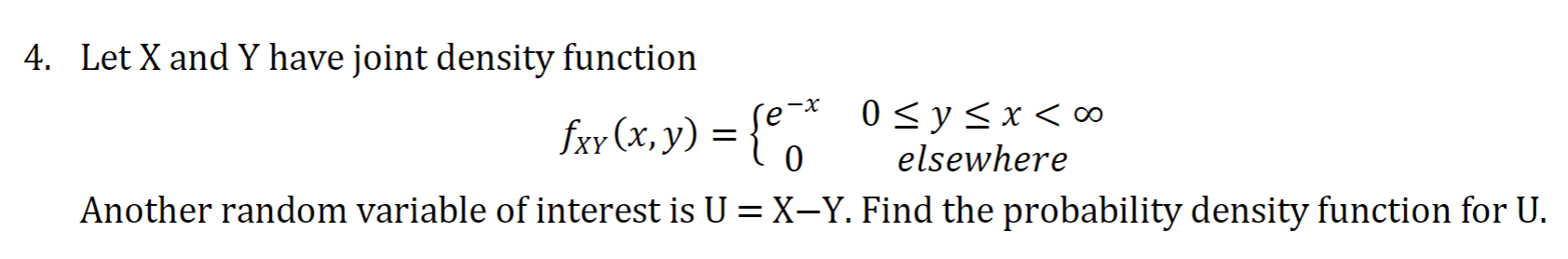4. Let X and Y have joint density function le-x 0 < y < x < 0 Jxy(x, y) = lo elsewhere Another random variable of interest is U=X–Y. Find the probability density function for U.

Free Homework App